• numpy数组切片与索引

万次阅读 2017-02-19 16:52:30
转自：...一、 如何切片索引： import numpy as np # Create the following rank 2 array with shape (3, 4) # [[ 1 2 3 4] # [ 5 6 7 8] # [ 9 1

一、 如何切片和索引：

import numpy as np

# Create the following rank 2 array with shape (3, 4)
# [[ 1  2  3  4]
#  [ 5  6  7  8]
#  [ 9 10 11 12]]
a = np.array([[1,2,3,4], [5,6,7,8], [9,10,11,12]])

# Use slicing to pull out the subarray consisting of the first 2 rows
# and columns 1 and 2; b is the following array of shape (2, 2):
# [[2 3]
#  [6 7]]
b = a[:2, 1:3]

# A slice of an array is a view into the same data, so modifying it
# will modify the original array.
print a[0, 1]   # Prints "2"
b[0, 0] = 77    # b[0, 0] is the same piece of data as a[0, 1]
print a[0, 1]   # Prints "77"

二、切片和索引容易搞混的地方（维度）

import numpy as np

# Create the following rank 2 array with shape (3, 4)
# [[ 1  2  3  4]
#  [ 5  6  7  8]
#  [ 9 10 11 12]]
a = np.array([[1,2,3,4], [5,6,7,8], [9,10,11,12]])

# Two ways of accessing the data in the middle row of the array.
# Mixing integer indexing with slices yields an array of lower rank,
# while using only slices yields an array of the same rank as the
# original array:
row_r1 = a[1, :]    # Rank 1 view of the second row of a
row_r2 = a[1:2, :]  # Rank 2 view of the second row of a
print row_r1, row_r1.shape  # Prints "[5 6 7 8] (4,)"  一维
print row_r2, row_r2.shape  # Prints "[[5 6 7 8]] (1, 4)" 二维

# We can make the same distinction when accessing columns of an array:
col_r1 = a[:, 1]
col_r2 = a[:, 1:2]
print col_r1, col_r1.shape  # Prints "[ 2  6 10] (3,)" 一维
print col_r2, col_r2.shape  # Prints "[[ 2]
#          [ 6]
#          ] (3, 1)" 二维

三、索引为数值时

import numpy as np

a = np.array([[1,2], [3, 4], [5, 6]])

# An example of integer array indexing.
# The returned array will have shape (3,) and
print a[[0, 1, 2], [0, 1, 0]]  # Prints "[1 4 5]"

# The above example of integer array indexing is equivalent to this:
print np.array([a[0, 0], a[1, 1], a[2, 0]])  # Prints "[1 4 5]"

# When using integer array indexing, you can reuse the same
# element from the source array:
print a[[0, 0], [1, 1]]  # Prints "[2 2]"  索引为（0,1） （0，1）

# Equivalent to the previous integer array indexing example
print np.array([a[0, 1], a[0, 1]])  # Prints "[2 2]"

import numpy as np

# Create a new array from which we will select elements
a = np.array([[1,2,3], [4,5,6], [7,8,9], [10, 11, 12]])

print a  # prints "array([[ 1,  2,  3],
#                [ 4,  5,  6],
#                [ 7,  8,  9],
#                [10, 11, 12]])"

# Create an array of indices
b = np.array([0, 2, 0, 1])

# Select one element from each row of a using the indices in b
print a[np.arange(4), b]  # Prints "[ 1  6  7 11]"

# Mutate one element from each row of a using the indices in b
a[np.arange(4), b] += 10

print a  # prints "array([[11,  2,  3],
#                [ 4,  5, 16],
#                [17,  8,  9],
#                [10, 21, 12]])

四、索引为布尔型时

import numpy as np

a = np.array([[1,2], [3, 4], [5, 6]])

bool_idx = (a > 2)  # Find the elements of a that are bigger than 2;
# this returns a numpy array of Booleans of the same
# shape as a, where each slot of bool_idx tells
# whether that element of a is > 2.

print bool_idx      # Prints "[[False False]
#          [ True  True]
#          [ True  True]]"

# We use boolean array indexing to construct a rank 1 array
# consisting of the elements of a corresponding to the True values
# of bool_idx
print a[bool_idx]  # Prints "[3 4 5 6]"

# We can do all of the above in a single concise statement:
print a[a > 2]     # Prints "[3 4 5 6]"

展开全文ndarray 切片 索引 numpy
• numpy数组切片传入索引与删除指定索引的行列 最近准备数据集，需要用到两个numpy的技巧，记录一下： 数组中切片传入索引 a = np.arange(20).reshape((4,5)) print(a) b = a[[1,2,3,0],[2,3,2,0]] print(b) [[ 0 1 2...

numpy数组切片传入索引与删除指定索引的行列

最近准备数据集，需要用到两个numpy的技巧，记录一下：

数组中切片传入索引

a = np.arange(20).reshape((4,5))
print(a)
b = a[[1,2,3,0],[2,3,2,0]]
print(b)

[[ 0  1  2  3  4]
[ 5  6  7  8  9]
[10 11 12 13 14]
[15 16 17 18 19]]

[ 7 13 17 0]

第一个列表代表的是点的行，第二个列表代表的是点的列

删除指定索引的行列

index = [1,3,]
value = np.delete(a,index,0)
print(value)

[[ 0  1  2  3  4]
[10 11 12 13 14]]

np.delete(array,obj,axis) 第三个参数0或1代表行或列

展开全文numpy python
• Numpy数组索引与切片 1. ndarray对象的内容可以通过索引或切片来获取和修改，就像 Python 的内置容器对象一样。 2. 一维数组:一维数组比较简单,看起来和python列表很类似。 3. 二维数组:每个索引值对应的...

Numpy数组索引与切片

1. ndarray对象的内容可以通过索引或切片来获取和修改，就像 Python 的内置容器对象一样。
2. 一维数组:一维数组比较简单,看起来和python列表很类似。
3. 二维数组:每个索引值对应的元素不在是一个值，而是一个一维数组
4. 多维数组
import numpy as np
a = np.arange(0,9)
print('一维数组操作')
print(a)
print('索引：',a)
print('切片：',a[2:5])
a[2:5]=88
print('赋值：',a)
print('*'*30)

print('二维数组操作')
b = a.reshape(3,3)
print(b)
print('索引：获取的一维数组',b) #获取的一个一维数组
print('分割列表去获取的某一个值',b[2,2]) #获取的某一个值
print('通过递归的方式获取的某一个值',b) #获取的某一个值
print('按列获取切片：',b[:,2])
print('按行获取切片：',b[2,:])
print('*'*30)

print('多维数组操作')
c = np.arange(1,19).reshape(3,3,2)
print(c)
print('索引：获得一个二维数组 获取第1层',c)
print('索引：获得一个一维数组 获取第1层的第1行',c[2,2])
print('索引：获得一个值',c[2,2,1])
一维数组操作
[0 1 2 3 4 5 6 7 8]
索引： 2
切片： [2 3 4]
赋值： [ 0  1 88 88 88  5  6  7  8]
******************************
二维数组操作
[[ 0  1 88]
[88 88  5]
[ 6  7  8]]
索引：获取的一维数组 [6 7 8]
分割列表去获取的某一个值 8
通过递归的方式获取的某一个值 8
按列获取切片： [88  5  8]
按行获取切片： [6 7 8]
******************************
多维数组操作
[[[ 1  2]
[ 3  4]
[ 5  6]]

[[ 7  8]
[ 9 10]
[11 12]]

[[13 14]
[15 16]
[17 18]]]
索引：获得一个二维数组 获取第1层 [[13 14]
[15 16]
[17 18]]
索引：获得一个一维数组 获取第1层的第1行 [17 18]
索引：获得一个值 18
******************************

Numpy布尔索引和花式索引

1. **布尔索引**:指的是一个由布尔值组成的数组可以作为一个数组的索引，返回的数据为True值对应位置的值。**布尔值数组长度必须和数组轴索引长度一致**。
2. **花式索引**:指的是用整数数组进行数据索引。根据索引数组的值作为目标数组的某个轴的下标来取值。对于使用一维整型数组作为索引，如果目标是一维数组，那么索引的结果就是对应位置的元素；如果目标是二维数组，那么就是对应下标的行
花式索引和切片不一样，它总是将数据复制到一个新的数组中。！！！`
import numpy as np
a = np.arange(1,10).reshape(3,3)
np.random.shuffle(a)
print(a)
print('布尔索引先返回一个true、false类型数据',a>5)
print('在根据结果返回元素值为True的元素',a[a>5],end='\n**************\n')

print('花式索引',end='\n**************\n')
print('花式索引',a[[0,2]])
print('花式索引',a[[-1,-2]])
print('花式索引:取出(0,2)，(2,1)，(1,2)----',a[[0,2,1],[2,1,2]])
[[4 5 6]
[7 8 9]
[1 2 3]]
布尔索引先返回一个true、false类型数据 [[False False  True]
[ True  True  True]
[False False False]]
在根据结果返回元素值为True的元素 [6 7 8 9]
**************
花式索引
**************
花式索引 [[4 5 6]
[1 2 3]]
花式索引 [[1 2 3]
[7 8 9]]
花式索引:取出(0,2)，(2,1)，(1,2)---- [6 2 9]
展开全文python numpy java 数据结构 列表
• NumPy 是一个 Python 包。 它代表 “Numeric Python”。它是一个由多维数组对象和用于处理数组的例程集合组成的库。这篇文章主要介绍了python numpy 数组索引切片,需要的朋友可以参考下
• 一、一维数组索引与切片 1.数组的索引是从0开始的； 2.数组脚标由右向左是从-1开始的，每向左一位数字减1 3.切片是[m,n)(即左闭右开)的区间； 4.将标量复制给切片，会广播到切片的整个区域。 二、二维数组 1....

一、一维数组索引与切片
1.数组的索引是从0开始的；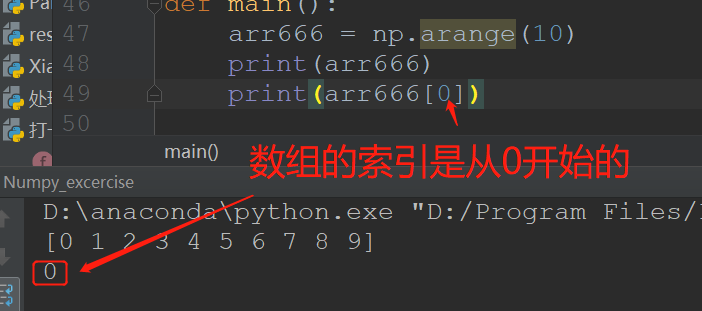2.数组脚标由右向左是从-1开始的，每向左一位数字减1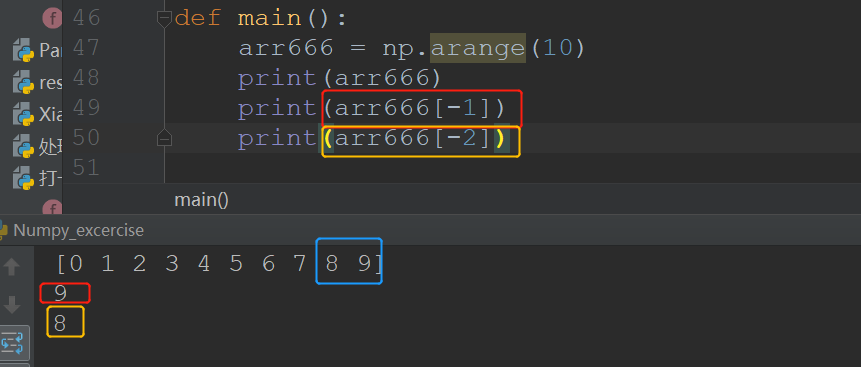3.切片是[m,n)(即左闭右开)的区间；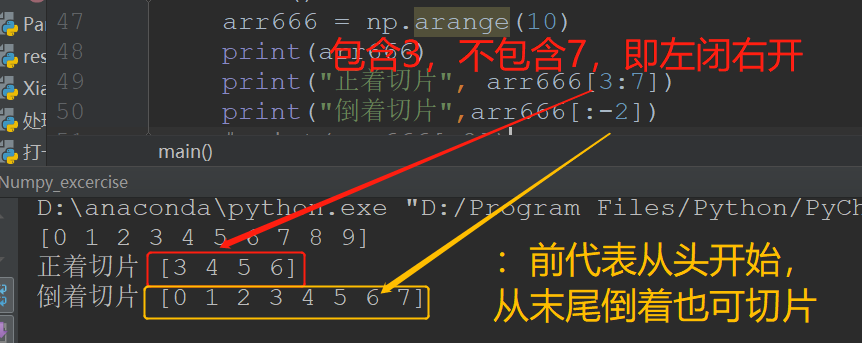4.将标量复制给切片，会广播到切片的整个区域。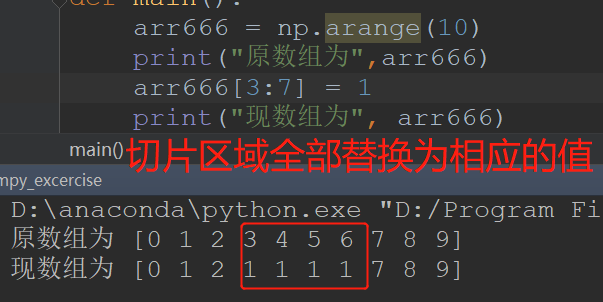二、二维数组
1.array_name:第一维脚标（索引值）是0的元素是一个数组；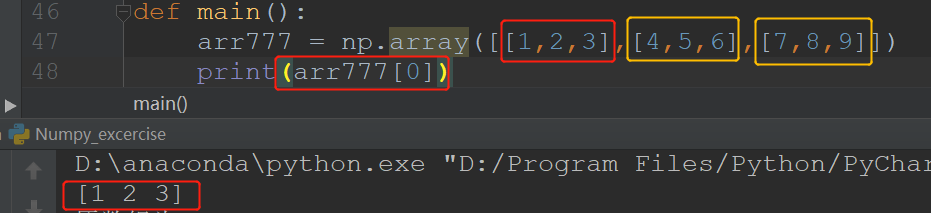2.array_name[m,n]:表示第一维脚标m，第二维为n的元素（或理解为m+1行，n+1列的元素）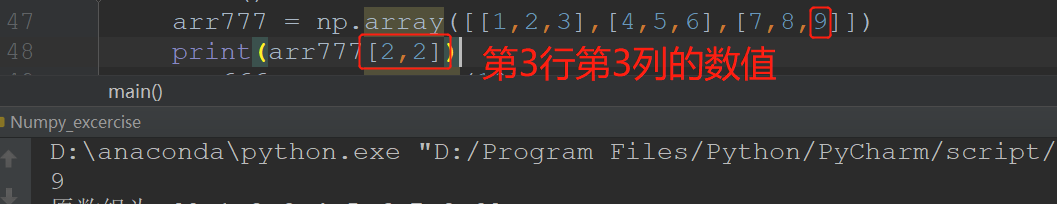3.array_name[：n]：选取前n行（未n-1，是因为行是从0开始的）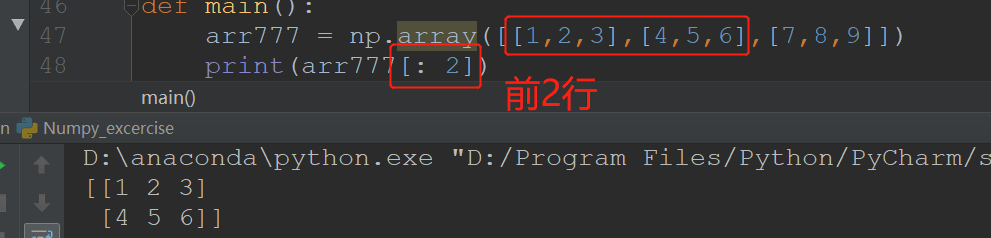4.array_name[m：n,x：y]：选取第一维度脚标第m到n-1行，选取第二维度脚标x到y-1行重叠的区域。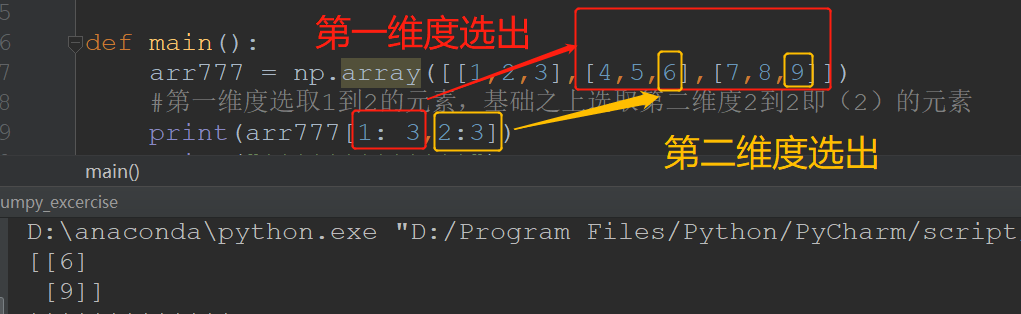展开全文• 一维数组切片索引与列表list是一样的，这里着重介绍二维数组。 1、递归方式 1.1 递归索引 import numpy as np arr = np.random.randint(0,10,(3,5)) print(arr) print(arr) [[4 3 ...numpy 索引 python
• Numpy数组的切片、索引和运算ndarray数组的切片和索引基础索引一维数组切片索引二维数组切片索引布尔索引一维数组的布尔索引二维数组的布尔索引神奇索引一维数组的神奇索引二维数组的神奇索引ndarray对象的运算...numpy python
• 1、切片 a = np.arange(10) s = slice(2,7,2) # 从索引 2 开始到索引 7 停止，间隔为2 print (a[s]) # [2 4 6] # 也可以通过冒号分隔切片参数 start:stop:step 来进行切片操作 a = np.arange(10) b = a[2:7:2] # 从...python numpy
• 1.概述今天我们来讲一下Numpy数组索引与切片,numpy数组索引与切片和Python中的切片与索引的作用相同,可以快速的取出数据,进行下一步的运用或者查看,但是两种切片还有一些不同的地方。另外我们可以通过数组的变形...
• Python中numpy数组索引问题，细节探究，详细讲解
• Python中numpy数组切片

千次阅读 2020-07-23 16:10:01
Python中符合切片并且常用的有：列表，字符串，元组。 下面那列表来说明，其他的也是一样的。 格式：[开头：结束：步长] 开头：当步长>0时，不写默认0。当步长<0时，不写默认-1 结束：当步长>0时，不写默认...python numpy
• numpy数组索引切片基本切片操作>>> import numpy as np>>> arr=np.arange(10)>>> arrarray([0, 1, 2, 3, 4, 5, 6, 7, 8, 9])>>> arr5>>> arr[5:8]array([5, 6,...
• 1.Python3中切片的定义 切片的语法表达式为：[start_index : end_...切片操作是指按照步长，截取从起始索引到结束索引，但不包含结束索引（也就是结束索引减1）的所有元素。 python3支持切片操作的数据类型有list...Numpy Python 数据分析
• numpy数组切片操作 通过冒号分隔切片参数 start:stop:step 来进行切片操作： 冒号 : 的解释：如果只放置一个参数，如 ，将返回索引相对应的单个元素。如果为 [2:]，表示从该索引开始以后的所有项都将被提取。...
• 你有这样的陈述：In : x[0:-1]这种索引方式意味着“从第一行开始到最后一行(不包括)”。所以我们得到了第一排。在^{pr2}\$但是，当你这样做的时候：In : x[1:-1]Out: array([], shape=(0, 5), dtype=int...
• NumPy 三维数组以及高维数组切片和索引

千次阅读 多人点赞 2020-09-04 15:55:47
1,Indexing and slicing numpy arrays https://www.pythoninformer.com/python-libraries/numpy/numpy-and-images/ In this section we will look at indexing and slicing. These work in a similar way to ...
• 一. 索引 1. 普通索引 ① 一维数组索引 ② 二维数组索引 ③ 三维数组索引 ...2. 布尔索引 ...3. 花式索引 ...二 ....1. 一维数组切片 2. 二维数组切片 3. 三维数组切片 三. 广播 ...
• 文章目录数组索引普通索引一维数组索引二维数组索引三维数组索引布尔索引花式索引数组切片一维数组切片二维数组切片三维数组切片数组元素重新赋值,语法，先筛选，再赋值矢量计算广播 数组索引 普通索引 一维数组索引...numpy
• 注：最近在看代码，有好多关于numpy库的函数，尤其对于numpy数组，什么[:,2]、[-1:,0:2]...numpy数组切片操作 通过冒号分隔切片参数 start:stop:step 来进行切片操作： 形如： b = a[2:7:2] # 从索引 2 开始到索引 7...numpy 数组切片
• 获取行或列【2×03】布尔索引【2×04】花式索引【3×00】NumPy 切片【4×00】NumPy 数组运算以及广播原则【7×00】数组的拼接元素的添加【7×01】将数组转换成列表，拼接完成再转换成数组【7×02】numpy.append()...
• 一:数组的属性每个数组都有它的属性,可分为:ndim(数组的维度),shape(数组每个维度的大小),size(数组的总大小),dtype(数组数据的类型)二:数组索引和python列表一样,Numpy索引在一维数组中,也可以通过中括号重指定...
• 索引切片•3. 读取文件•4. 布尔型索引•5. 数组的运算•6. 常用函数举例NumPy 是 Numerical Python 的简称，是 Python 科学计算的核心包，也是高性能科学计算和数据分析的基础包。numpy特性：具备功能非常强大的...
• Numpy数组的索引与切片：取数组的特定行列

万次阅读 多人点赞 2018-11-24 23:32:33
主要介绍numpy数组索引的方式，如切片索引、数组索引python numpy 索引
• NumPy库–数组索引切片详解 1. 常用索引 获取某行的数据： # 1. 如果是一维数组 a1 = np.arange(0,29) print(a1) print(a1) # 获取下标为1的元素，结果为1 a1 = np.arange(0,24).reshape((4,6)) print(a1) ...python 数据分析
• import numpy as np 一、基本索引 获取单个元素 a = np.diag(np.arange(3)) print(a[1,1]) 1 获取一组元素 print(a) [0 1 0] 修改元素 a[2,1] = 10 print(a) [[ 0 0 0] [ 0 1 0] [ 0 10 2]...Numpy索引 切片 花式索引 多维数组
• Numpy数组的访问模式和python中的list相似，在多维的数组中使用, 进行区分： 在python的list 下： a = [1,2,4] print a[2:] 打印出：  这是一个数组，在Numpy的多维数组中也采用相同的模式进行数组的访问......